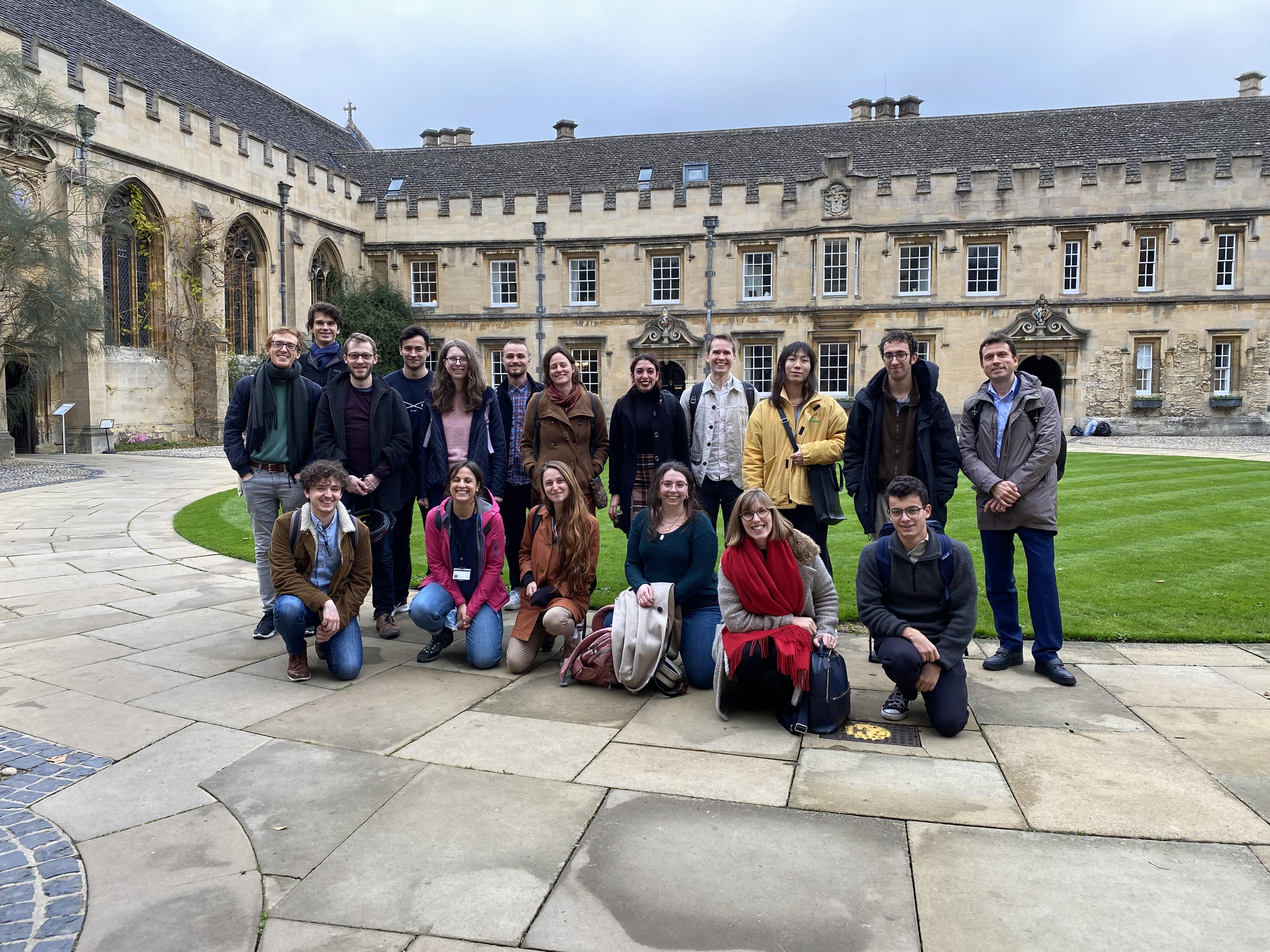# Algebraic Systems Biology

Much of our research is motivated by applications. We develop models and methods to study primarily biological and chemical systems; however, our work is also applied towards engineering, medical, physical and social problems. Such analysis often requires working with data.

Our research group uses mathematical and statistical techniques including numerical algebraic geometry, Bayesian statistics, computational topology, differential equations, linear algebra, network science, and optimisation, in order to solve interdisciplinary problems. Our research interests include applied algebraic geometry, algebraic statistics, dynamical systems, topological data analysis, cellular signaling, chemical reaction network theory, mathematical and systems biology.

The research group is led by Heather Harrington. See our members page for more details. We are mathematicians working at the interface of theoretical, applied, and data science.